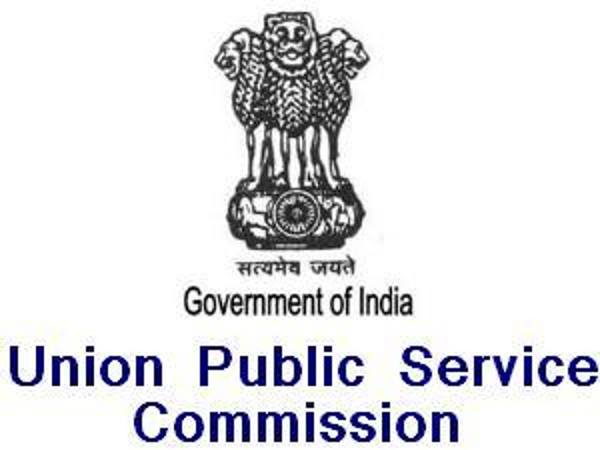# Last-minute Tips To Crack Mathematics In UPSC NDA & NA

By Sashikanth

National Defence Academy & Naval Academy (NDA & NA), conducted by the Union Public Service Commission (UPSC), is one amongst the popular defence examinations in India. Annually, the UPSC conducts NDA & NA (I) and (II) examinations in the month of April and September for admission to the Army, Navy and Air Force wings. Here are the preparation tips for Mathematics in NDA & NA exam.

## NDA & NA Scheme of Examination

 Subject Duration Marks Mathematics 150 Minutes 300 General Ability Test 150 Minutes 600 Total 900 SSB Interview 900

The examination will consist of objective-type questions only. The exam is conducted offline. The UPSC will fix the qualifying marks for all the subjects in the examination. Candidates who qualify in the written test will be called for the SSB interview.Preparation Tips For NDA Exam

## NDA & NA Mathematics Preparation Tips

• Compared to the general ability test, the mathematics paper has less weightage but plays a key role in shortlisting the candidates. The mathematics subject is not a hard nut to crack, if you are good at class 11 and 12 NCERT mathematics textbooks. Here are the important tips to crack the paper-I, i.e., mathematics.
• The revision part is very crucial for mathematics. On the last day of your preparations, devote at least three hours for going through the formulae.
• Never get confused while converting numbers from the decimal system to the binary system.
• The trigonometry questions are usually tricky. So be clear in the concepts such as properties of triangles and inverse trigonometric functions.
• The questions related to analytical geometry of two and three dimensions consume a lot of time. Hence, keep this for the end.
• Most of the concepts in differential calculus are important, however, give importance to algebraic operations on continuous functions and applications of derivatives in problems of maxima and minima.
• The vector algebra is the most important unit in the syllabus. Give cornerstone to vector product or cross product of two vectors.
• The statistics part is easy to crack once you've got the logic, but be careful while taking the figures. A single mistake may cost you a lot.
• In probability, the elementary theorems, Bayes' theorem and binomial distribution are important. However, don't give more than two minutes to solve the questions.
• Practise is the only success formula to score well in mathematics. In this final week, practise at least for two hours daily.

You might be pretty satisfied with our mathematics tips. Acing this paper alone isn't enough for you to land a good score in the examination. The most important paper in NDA & NA is the general ability test. Explore the section-wise tips for the general ability test with a single click.

Subscribe Now

--Or--
Select a Field of Study
Select a Course
Select UPSC Exam
Select IBPS Exam
Select Entrance Exam